Next: Properties of Fourier transforms Up: Fourier analysis of periodic Previous: Periodicity of the Fourier   Contents   Index

## Fourier transform as additive synthesis

Now consider an arbitrary signalthat repeats everysamples. (Previously we had assumed thatcould be obtained as a sum of sinusoids, and we haven't yet found out whether every periodiccan be obtained that way.) Let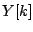denote the Fourier transform offor: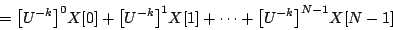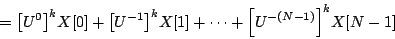In the second version we rearranged the exponents to show thatis a sum of complex sinusoids, with complex amplitudesand frequenciesfor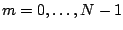. In other words,can be considered as a Fourier series in its own right, whoseth component has strength. (The expressionmakes sense becauseis a periodic signal). We can also express the amplitude of the partials ofin terms of its own Fourier transform. Equating the two gives:This means in turn thatcan be obtained by summing sinusoids with amplitudes. Setting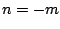gives:This shows that any periodiccan indeed be obtained as a sum of sinusoids. Further, the formula explicitly shows how to reconstructfrom its Fourier transform, if we know its value for the integers.Next: Properties of Fourier transforms Up: Fourier analysis of periodic Previous: Periodicity of the Fourier   Contents   Index
Miller Puckette 2006-12-30